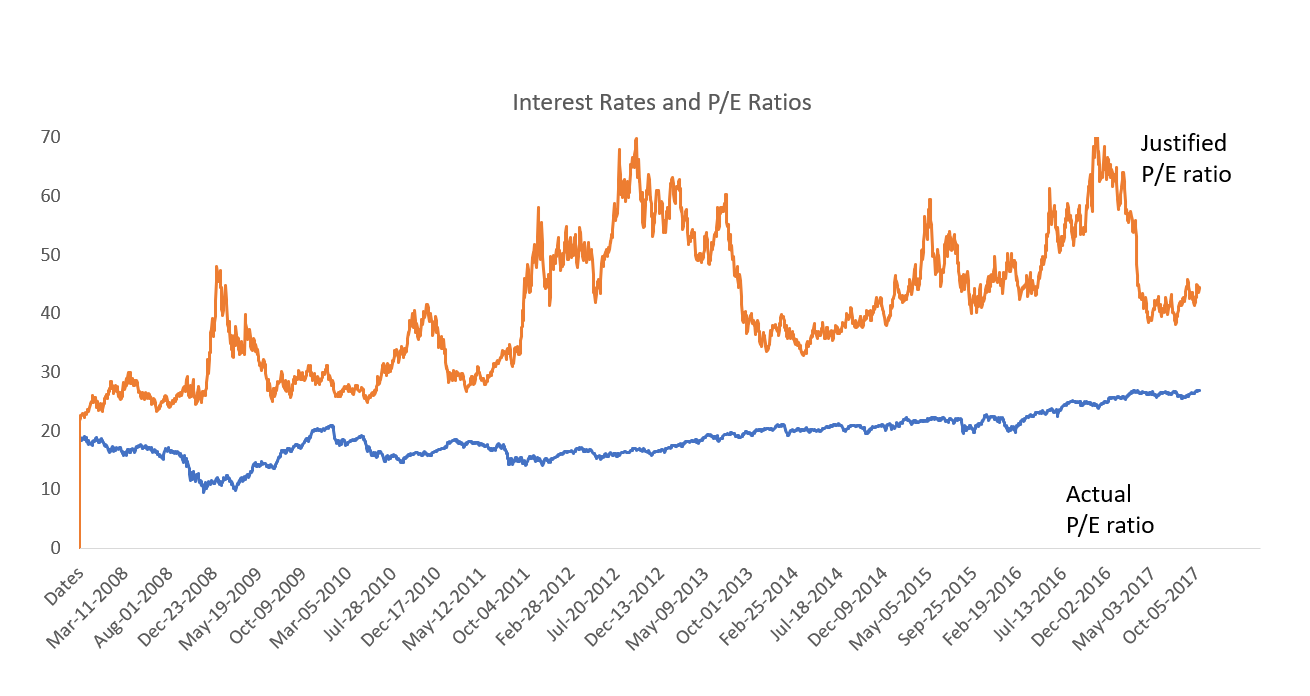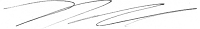The bears have great arguments in the current market. Among the most interesting is that stocks are overvalued. But with interest rates where they are, stocks are deeply undervalued.

The chart below shows the actual price-to-earnings (P/E) ratio of the S&P 500 and the justified P/E ratio, which is the fair value of the ratio based on interest rates.The current P/E ratio is 26.9. That’s higher than average, and that has the bears worried.

Legendary investor Warren Buffett’s teacher, Ben Graham, taught that the proper P/E ratio depended on interest rates. He wrote that the P/E ratio should be equal to the inverse of the long-term interest rate.

This sounds confusing, but it’s easy to understand. That relationship is based on the idea that stocks and bonds are alternative investments. Graham developed this idea in the 1930s, when stocks and bonds were direct alternatives.

Graham believed investors should buy stocks only when the company earned more than bonds paid. Bonds pay an interest rate. The equivalent measure for stocks is the earnings yield.

The earnings yield is the earnings per share divided by the stock’s price. Mathematically, that’s the E/P ratio. It’s also called the justified P/E ratio. This lets us determine what the right ratio for stocks is.

If we know the current interest rate, dividing that rate by one tells us what the fair value of the P/E ratio should be. With low rates, the fair value of the P/E ratio is high. In fact, it’s about 44 right now.

It looks like rates are rising. As this happens, the fair value of the P/E ratio, or the justified P/E ratio, will fall. If 10-year Treasury rates rise by 0.5%, the justified P/E ratio falls to about 35.

This all means stocks have significant upside potential. Low interest rates and high earnings growth point to higher prices in the stock market.

There’s no need to worry about valuation for now.

Regards,Michael Carr, CMT